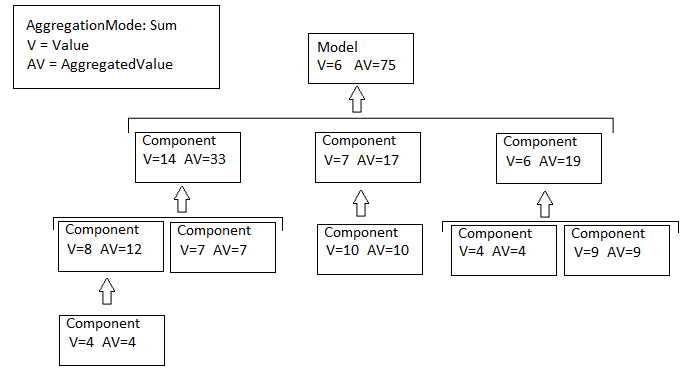## Model Metric Data Aggregation

You can better understand the size, complexity, and readability of a model and its components by analyzing aggregated model metric data. Aggregated metric data is available in the `AggregatedValue` and `AggregatedMeasures` properties of an `slmetric.metric.Result` object. The `AggregatedValue` property aggregates the metric scalar values. The `AggregatedMeasures` property aggregates the metric measures (that is, the detailed information about the metric values).

### How Model Metric Aggregation Works

The implementation of a model metric defines how a metric aggregates data across a component hierarchy. For MathWorks model metrics, the `slmetric.metric.Metric` class defines model metric aggregation. This class includes the `AggregationMode` property, which has these options:

• `Sum`: Returns the sum of the `Value` property and the `Value` properties of its children components across the component hierarchy. Returns the sum of the `Meaures` property and the `Measures` properties of its children components across the component hierarchy.

• `Max`: Returns the maximum of the `Value` property and the `Value` properties of its children components across the component hierarchy. Returns the maximum of the `Measures` property and the `Measures` properties of its children components across the component hierarchy.

• `None`: No aggregation of metric values.

You can find descriptions of MathWorks model metrics and their `AggregationMode` property setting in Model Metrics. For custom metrics, as part of the `algorithm` method, you can define how the metric aggregates data. For more information, see Create a Custom Model Metric for Nonvirtual Block Count.

This diagram shows how the software aggregates metric data across the components of a model hierarchy. The parent model is at the top of the hierarchy. The components can be the following:

• Model

• Subsystem block

• Chart

• MATLAB function block

• Protected model### Access Aggregated Metric Data

This example shows how to collect metric data programmatically in the metric engine, and then access aggregated metric data.

1. Load the `sldemo_applyVarStruct` model.

```model = 'sldemo_applyVarStruct'; open(model); load_system(model);```
2. Create an `slmetric.Engine` object and set the analysis root.

```metric_engine = slmetric.Engine(); setAnalysisRoot(metric_engine,'Root',model,'RootType','Model');```

3. Collect data for the Input output model metric.

`execute(metric_engine,'mathworks.metrics.IOCount');`
4. Get the model metric data that returns an array of `slmetric.metric.ResultCollection` objects, `res_col`. Specify the input argument for `AggregationDepth`.

```res_col = getMetrics(metric_engine,'mathworks.metrics.IOCount',... 'AggregationDepth','All');```

The `AggregationDepth` input argument has two options: `All` and `None`. If you do not want the `getMetrics` method to aggregate measures and values, specify `None`.

5. Display the results.

```metricData ={'MetricID','ComponentPath','Value',... 'AggregatedValue','Measures','AggregatedMeasures'}; cnt = 1; for n=1:length(res_col) if res_col(n).Status == 0 results = res_col(n).Results; for m=1:length(results) disp(['MetricID: ',results(m).MetricID]); disp([' ComponentPath: ',results(m).ComponentPath]); disp([' Value: ',num2str(results(m).Value)]); disp([' Aggregated Value: ',num2str(results(m).AggregatedValue)]); disp([' Measures: ',num2str(results(m).Measures)]); disp([' Aggregated Measures: ',... num2str(results(m).AggregatedMeasures)]); metricData{cnt+1,1} = results(m).MetricID; metricData{cnt+1,2} = results(m).ComponentPath; metricData{cnt+1,3} = results(m).Value; tdmetricData{cnt+1,4} = results(m).Measures; metricData{cnt+1,5} = results(m).AggregatedMeasures; cnt = cnt + 1; end else disp(['No results for:',res_col(n).MetricID]); end disp(' '); end```

Here are the results:

```MetricID: mathworks.metrics.IOCount ComponentPath: sldemo_applyVarStruct Value: 3 Aggregated Value: 5 Measures: 1 2 0 0 Aggregated Measures: 3 2 0 0 MetricID: mathworks.metrics.IOCount ComponentPath: sldemo_applyVarStruct/Controller Value: 4 Aggregated Value: 4 Measures: 3 1 0 0 Aggregated Measures: 3 1 0 0 MetricID: mathworks.metrics.IOCount ComponentPath: sldemo_applyVarStruct/Aircraft Dynamics Model Value: 5 Aggregated Value: 5 Measures: 3 2 0 0 Aggregated Measures: 3 2 0 0 MetricID: mathworks.metrics.IOCount ComponentPath: sldemo_applyVarStruct/Dryden Wind Gust Models Value: 2 Aggregated Value: 2 Measures: 0 2 0 0 Aggregated Measures: 0 2 0 0 MetricID: mathworks.metrics.IOCount ComponentPath: sldemo_applyVarStruct/Nz pilot calculation Value: 3 Aggregated Value: 3 Measures: 2 1 0 0 Aggregated Measures: 2 1 0 0 MetricID: mathworks.metrics.IOCount ComponentPath: sldemo_applyVarStruct/More Info2 Value: 0 Aggregated Value: 0 Measures: 0 0 0 0 Aggregated Measures: 0 0 0 0```

For the Input output metric, the `AggregationMode` is `Max`. For each component, the `AggregatedValue` and `AggregatedMeasures` properties are the maximum number of inputs and outputs of itself and its children components. For example, for `sldemo_applyVarStruct`, the `AggregatedValue` property is `5`, which is the `sldemo_applyVarStruct/Aircraft Dynamics Model` component value.